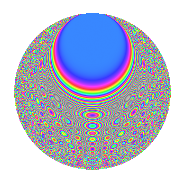# Properties

 Label 525.4.bcLevel $525$ Weight $4$ Character orbit 525.bc Rep. character $\chi_{525}(82,\cdot)$ Character field $\Q(\zeta_{12})$ Dimension $288$ Sturm bound $320$

# Related objects

## Defining parameters

 Level: $$N$$ $$=$$ $$525 = 3 \cdot 5^{2} \cdot 7$$ Weight: $$k$$ $$=$$ $$4$$ Character orbit: $$[\chi]$$ $$=$$ 525.bc (of order $$12$$ and degree $$4$$) Character conductor: $$\operatorname{cond}(\chi)$$ $$=$$ $$35$$ Character field: $$\Q(\zeta_{12})$$ Sturm bound: $$320$$

## Dimensions

The following table gives the dimensions of various subspaces of $$M_{4}(525, [\chi])$$.

Total New Old
Modular forms 1008 288 720
Cusp forms 912 288 624
Eisenstein series 96 0 96

## Trace form

 $$288q + 4q^{7} - 168q^{8} + O(q^{10})$$ $$288q + 4q^{7} - 168q^{8} - 112q^{11} + 2080q^{16} + 48q^{21} - 624q^{22} + 64q^{23} - 1824q^{26} - 628q^{28} + 1056q^{31} + 672q^{32} + 108q^{33} - 10368q^{36} + 552q^{37} + 4044q^{38} + 1068q^{42} - 720q^{43} - 680q^{46} - 240q^{47} - 672q^{51} - 4644q^{52} - 1728q^{53} - 9792q^{56} - 1392q^{57} - 512q^{58} - 1296q^{61} + 288q^{63} + 8208q^{66} + 3784q^{67} + 8844q^{68} + 256q^{71} + 756q^{72} + 3312q^{73} + 6600q^{77} + 1200q^{78} + 11664q^{81} - 6084q^{82} - 7784q^{86} - 5652q^{87} - 4844q^{88} - 11976q^{91} + 2152q^{92} - 1368q^{93} - 20184q^{98} + O(q^{100})$$

## Decomposition of $$S_{4}^{\mathrm{new}}(525, [\chi])$$ into newform subspaces

The newforms in this space have not yet been added to the LMFDB.

## Decomposition of $$S_{4}^{\mathrm{old}}(525, [\chi])$$ into lower level spaces

$$S_{4}^{\mathrm{old}}(525, [\chi]) \cong$$ $$S_{4}^{\mathrm{new}}(35, [\chi])$$$$^{\oplus 4}$$$$\oplus$$$$S_{4}^{\mathrm{new}}(105, [\chi])$$$$^{\oplus 2}$$$$\oplus$$$$S_{4}^{\mathrm{new}}(175, [\chi])$$$$^{\oplus 2}$$

## Hecke characteristic polynomials

There are no characteristic polynomials of Hecke operators in the database